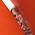## Saturday, July 19, 2014

### The Rise and Fall of the name of Jennifer

A recent fascinating post by Analysis at Large got me interested in naming trends analysis. The original post used Social Security data by state to map out the more frequent names by state. While this sounded interesting it turned out a single name (you can read the post yourself to discover that name) dominated the map for all but two states (California and Nevada) which had Jennifer as their most frequent name.  The male name distribution was more heterogeneous but still not very informative.

Looking at the data from SS I wanted to see not only what were the most popular names since 1880 (as the previous post had found) but also how the popularity of the names changed over time.  First looking at the Figure 1 and 2 show the most popular Male and Female names in terms of the total number of Americans named a particular name. For males there were over 5 million Johns and James and for females there was a little over 4 million Marys.

Figures 3 and 4 show how the top 7 male and female names have risen and fallen in count values over time. It is interesting to note the distinctly different pattern among male names as female names.  Male name popularity among the top 7 seems generally unimodal while female names have distinctly different peaks at different places.

In order to cut down on extraneous noise caused from rises and falls of birth rates I also included measurements of what proportion of the total names listed (in the entire SS list) each of the top 7 names represented (Figure 5 and 6).  I find these figures much more interesting as they show how some names such as John and William which extremely popular in the 1880s have since fallen dramatically in popularity. Likewise among women the name Mary has fallen almost constantly from a unique peak to a dominative level among female names.  It is interesting that among women female names seem to peak with high popularity from very low levels of usage such as the names Jennifer, Margarette, Linda, and Barbara then once again fall in popularity.

Finally in an attempt to get at the right most tails of these trends which uniformly seem to be trending down, I ranked the names among the total number of instances per year for each name. Figure 7 and 8 show the results.  In both figures the y axis is scaled by log 10. For men the name Micheal is particularly interesting with very low ranking on usage in the early 20th century and emerging as the most popular name in the decades between 1960 and 2000. Even more interesting, the among the female names the name Jennifer first appeared around 1918 with a usage ranking around 5000 only to steadily gain popularity until being the most popular name is use in the 1970s to early 1980s.  Other popular names such as Patricia, Margette, and Barbara followed similar patterns though none so stark.

The total rankings of female names confirms a lose in popularity of traditionally popular names with perhaps the slight exception of Elizabeth which while never extremely popular has managed to stay around the top 10 most popular names in recent decades.  Among male names there does not seem to be as much of a faddish behavior with all of the 7 names observed remaining within the top 60 names in total of those chosen.

To see how these tables are produced you may find my R code below the figures or on github.

Figure 1
Figure 2

Figure 3
Figure 4
Figure 5
Figure 6
Figure 7
Figure 8
R Code
```require(plyr)
require(ggplot2)
require(scales)

# http://www.ssa.gov/oact/babynames/names.zip
setwd("C:/Data/SS-names/")
files<-list.files()
files<-files[grepl(".txt",files)]

namedata <- matrix(0,ncol=4,nrow=0)

for (i in 1:length(files))
namedata<-rbind(namedata,

colnames(namedata)<-c("name","gender","count", "year")

dim(namedata)
# 1.8 million rows

Mdata<-namedata[namedata\$gender=="M",]
Fdata<-namedata[namedata\$gender=="F",]

Msums <- ddply(Mdata, .(name), summarize, sum=sum(count))
Fsums <- ddply(Fdata, .(name), summarize, sum=sum(count))

nrow(Msums); nrow(Fsums)
# There are 38601 male names and 64089 female names

Morder <- Msums[order(Msums[,2], decreasing = TRUE),]
Forder <- Fsums[order(Fsums[,2], decreasing = TRUE),]

c <- ggplot(Morder[1:20,], aes(x = name, y = sum, size=sum))
c + geom_point() + coord_flip() + theme(legend.position="none")+
ggtitle("20 Most Popular Male Names Since 1880")+
xlab("")+```
` scale_y_continuous(name="Names Recorded With Social Security Administration",  `
```labels = comma)
# Figure 1

c <- ggplot(Forder[1:20,], aes(x = name, y = sum, size=sum))
c + geom_point() + coord_flip() + theme(legend.position="none")+
ggtitle("20 Most Popular Female Names Since 1880")+
xlab("")+```
` scale_y_continuous(name="Names Recorded With Social Security Administration",  `
```labels = comma)
# Figure 2

Mdata\$order <- Fdata\$torder <- NA # Create a variable for
Mdata\$prop <- Fdata\$prop <- NA

for (i in 1880:2013) {
Mdata[Mdata\$year==i, "torder"] <-
order(-Mdata[Mdata\$year==i, "count"])
Mdata[Mdata\$year==i, "prop"] <-
(Mdata[Mdata\$year==i, "count"])/
sum((Mdata[Mdata\$year==i, "count"]))
Fdata[Fdata\$year==i, "torder"] <-
order(-Fdata[Fdata\$year==i, "count"])
Fdata[Fdata\$year==i, "prop"] <-
(Fdata[Fdata\$year==i, "count"])/
sum((Fdata[Fdata\$year==i, "count"]))
}

top <- 7

Mrestricted <- Mdata[Mdata\$name%in%Morder[1:top,1],]
Frestricted <- Fdata[Fdata\$name%in%Forder[1:top,1],]

ggplot(Mrestricted, aes(x=year, y=count, group=name, color=name))+
geom_line(size=1)+scale_x_discrete(breaks=seq(1880,2010,20))

ggplot(Mrestricted, aes(x=year, y=prop, group=name, color=name))+
geom_line(size=1)+scale_x_discrete(breaks=seq(1880,2010,20))+
ylab("Proportion of Total Names")

ggplot(Mrestricted,
aes(x=year, y=torder, group=name, color=name, size=torder))+
geom_line()+scale_x_discrete(breaks=seq(1880,2010,20))+
ylab("Order of Total Names That Year (log10)")+scale_y_log10()

ggplot(Frestricted, aes(x=year, y=count, group=name, color=name))+
geom_line(size=1)+scale_x_discrete(breaks=seq(1880,2010,20))

ggplot(Frestricted, aes(x=year, y=prop, group=name, color=name))+
geom_line(size=1)+scale_x_discrete(breaks=seq(1880,2010,20))+
ylab("Proportion of Total Names")

ggplot(Frestricted,
aes(x=year, y=torder, group=name, color=name, size=torder))+
geom_line()+scale_x_discrete(breaks=seq(1880,2010,20))+
ylab("Order of Total Names That Year (log10)")+scale_y_log10()```
Created by Pretty R at inside-R.org

#### 1 comment:

1.Interesting post. I would be curious to see if the rise and fall of name usage over time follows a pattern with respect to demographics (e.g. age of parents), socio-economic characteristics of families (e.g. from low to high-income, or vice-versa), geographic location (e.g. spread from one part of the country to another)...